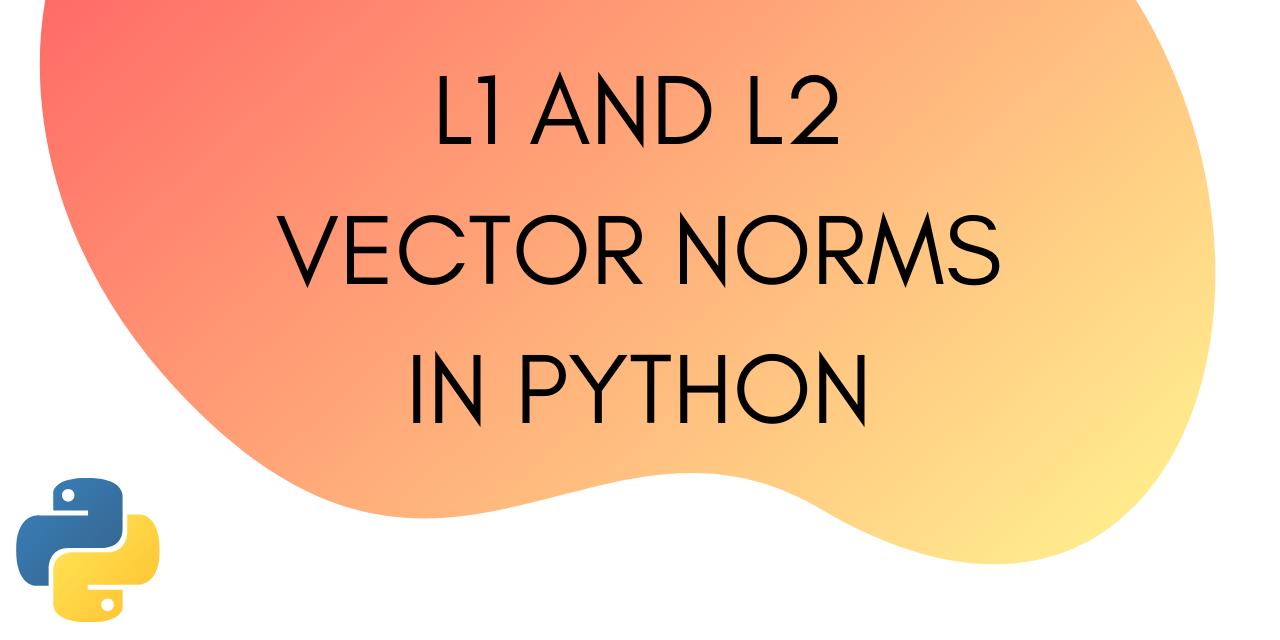# Norm of a Vector in Python - Steps for Calculation

Published on August 3, 2022By Jayant VermaWhile we believe that this content benefits our community, we have not yet thoroughly reviewed it. If you have any suggestions for improvements, please let us know by clicking the “report an issue“ button at the bottom of the tutorial.

The norm of a vector refers to the length or the magnitude of a vector. There are different ways to calculate the length. The norm of a vector is a non-negative value. In this tutorial, we will learn how to calculate the different types of norms of a vector.

Norm of a vector x is denoted as: ‖x

The norm of a vector is a measure of its distance from the origin in the vector space.

To calculate the norm, you can either use Numpy or Scipy. Both offer a similar function to calculate the norm.

In this tutorial we will look at two types of norms that are most common in the field of machine learning.

These are :

• L1 Norm
• L2 Norm

## How to Calculate the L1 Norm of a Vector?

L1 Norm of a vector is also known as the Manhattan distance or Taxicab norm. The notation for L1 norm of a vector x is ‖x‖1.

To calculate the norm, you need to take the sum of the absolute vector values.

Let’s take an example to understand this:

``````a = [1,2,3,4,5]
``````

For the array above, the L1 norm is going to be:

``````1+2+3+4+5 = 15
``````

Let’s take another example:

``````a = [-1,-2,3,4,5]
``````

The L1 norm of this array is :

``````|-1|+|-2|+3+4+5 = 15
``````

The L1 norm for both the vectors is the same as we consider absolute values while computing it.

### Python Implementation of L1 norm

Let’s see how can we calculate L1 norm of a vector in Python.

### Using Numpy

The Python code for calculating L1 norm using Numpy is as follows :

``````from numpy import array
from numpy.linalg import norm
arr = array([1, 2, 3, 4, 5])
print(arr)
norm_l1 = norm(arr, 1)
print(norm_l1)
``````

Output :

``````[1 2 3 4 5]
15.0
``````

Let’s try calculating it for the array with negative entries in our example above.

``````from numpy import array
from numpy.linalg import norm
arr = array([-1, -2, 3, 4, 5])
print(arr)
norm_l1 = norm(arr, 1)
print(norm_l1)
``````

Output :

``````[-1 -2  3  4  5]
15.0
``````

### Using Scipy

To calculate L1 using Scipy is not very different from the implementation above.

The code for same is:

``````from numpy import array
from scipy.linalg import norm
arr = array([-1, -2, 3, 4, 5])
print(arr)
norm_l1 = norm(arr, 1)
print(norm_l1)
``````

Output :

``````[-1 -2  3  4  5]
15.0
``````

The code is exactly similar to the Numpy one.

## How to Calculate L2 Norm of a Vector?

The notation for the L2 norm of a vector x is ‖x‖2.

To calculate the L2 norm of a vector, take the square root of the sum of the squared vector values.

Another name for L2 norm of a vector is Euclidean distance. This is often used for calculating the error in machine learning models.

The Root Mean square error is the Euclidean distance between the actual output of the model and the expected output.

The goal of a machine learning model is to reduce this error.

Let’s consider an example to understand it.

``````a = [1,2,3,4,5]
``````

The L2 norm for the above is :

``````sqrt(1^2 + 2^2 + 3^2 + 4^2 + 5^2) = 7.416
``````

L2 norm is always a positive quantity since we are squaring the values before adding them.

### Python Implementation

The Python implementation is as follows :

``````from numpy import array
from numpy.linalg import norm
arr = array([1, 2, 3, 4, 5])
print(arr)
norm_l2 = norm(arr)
print(norm_l2)
``````

Output :

``````[1 2 3 4 5]
7.416198487095663
``````

Here we can see that by default the norm method returns the L2 norm.

## Conclusion

This tutorial was about calculating L1 and L2 norms in Python. We used Numpy and Scipy to calculate the two norms. Hope you had fun learning with us!

Thanks for learning with the DigitalOcean Community. Check out our offerings for compute, storage, networking, and managed databases.Jayant Verma

author

#### Still looking for an answer?

Ask a questionSearch for more help

JournalDev
DigitalOcean EmployeeNovember 6, 2020

your article contradicts usual mathematical definitions - standard L1 is defined such as not to be less than zero. - you name L2 the square of the standard L2 , put units to your vector you’ll see is not the same see https://en.wikipedia.org/wiki/Norm\_(mathematics)

- Pablo Balonga

Click below to sign up and get \$200 of credit to try our products over 60 days!

### Popular Topics##### Hollie's Hub for Good

Working on improving health and education, reducing inequality, and spurring economic growth? We’d like to help.##### Become a contributor

You get paid; we donate to tech nonprofits.

##### Welcome to the developer cloud

DigitalOcean makes it simple to launch in the cloud and scale up as you grow – whether you’re running one virtual machine or ten thousand.© 2023 DigitalOcean, LLC.